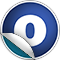scalaz

# Leibniz

#### object Leibniz extends LeibnizInstances with LeibnizFunctions

Source
Leibniz.scala
Linear Supertypes
Ordering
1. Alphabetic
2. By inheritance
Inherited
1. Leibniz
2. LeibnizFunctions
3. LeibnizInstances
4. AnyRef
5. Any
1. Hide All
2. Show all
Learn more about member selection
Visibility
1. Public
2. All

### Type Members

1. #### type ===[A, B] = Leibniz[⊥, ⊤, A, B]

`(A === B)` is a supertype of `Leibniz[L,H,A,B]`

### Value Members

1. #### final def !=(arg0: AnyRef): Boolean

Definition Classes
AnyRef
2. #### final def !=(arg0: Any): Boolean

Definition Classes
Any
3. #### final def ##(): Int

Definition Classes
AnyRef → Any
4. #### final def ==(arg0: AnyRef): Boolean

Definition Classes
AnyRef
5. #### final def ==(arg0: Any): Boolean

Definition Classes
Any
6. #### final def asInstanceOf[T0]: T0

Definition Classes
Any
7. #### def clone(): AnyRef

Attributes
protected[java.lang]
Definition Classes
AnyRef
Annotations
@throws( ... )
8. #### final def eq(arg0: AnyRef): Boolean

Definition Classes
AnyRef
9. #### def equals(arg0: Any): Boolean

Definition Classes
AnyRef → Any
10. #### def finalize(): Unit

Attributes
protected[java.lang]
Definition Classes
AnyRef
Annotations
@throws( classOf[java.lang.Throwable] )
11. #### def force[L, H >: L, A >: L <: H, B >: L <: H]: Leibniz[L, H, A, B]

Unsafe coercion between types.

Unsafe coercion between types. force abuses asInstanceOf to explicitly coerce types. It is unsafe, but needed where Leibnizian equality isn't sufficient

Definition Classes
LeibnizFunctions
12. #### final def getClass(): Class[_]

Definition Classes
AnyRef → Any
13. #### def hashCode(): Int

Definition Classes
AnyRef → Any
14. #### final def isInstanceOf[T0]: Boolean

Definition Classes
Any
15. #### implicit def leibniz: Category[===]

Definition Classes
LeibnizInstances
16. #### def lift[LA, LT, HA >: LA, HT >: LT, T[_ >: LA <: HA] >: LT <: HT, A >: LA <: HA, A2 >: LA <: HA](a: Leibniz[LA, HA, A, A2]): Leibniz[LT, HT, T[A], T[A2]]

We can lift equality into any type constructor

We can lift equality into any type constructor

Definition Classes
LeibnizFunctions
17. #### def lift2[LA, LB, LT, HA >: LA, HB >: LB, HT >: LT, T[_ >: LA <: HA, _ >: LB <: HB] >: LT <: HT, A >: LA <: HA, A2 >: LA <: HA, B >: LB <: HB, B2 >: LB <: HB](a: Leibniz[LA, HA, A, A2], b: Leibniz[LB, HB, B, B2]): Leibniz[LT, HT, T[A, B], T[A2, B2]]

We can lift equality into any type constructor

We can lift equality into any type constructor

Definition Classes
LeibnizFunctions
18. #### def lift3[LA, LB, LC, LT, HA >: LA, HB >: LB, HC >: LC, HT >: LT, T[_ >: LA <: HA, _ >: LB <: HB, _ >: LC <: HC] >: LT <: HT, A >: LA <: HA, A2 >: LA <: HA, B >: LB <: HB, B2 >: LB <: HB, C >: LC <: HC, C2 >: LC <: HC](a: Leibniz[LA, HA, A, A2], b: Leibniz[LB, HB, B, B2], c: Leibniz[LC, HC, C, C2]): Leibniz[LT, HT, T[A, B, C], T[A2, B2, C2]]

We can lift equality into any type constructor

We can lift equality into any type constructor

Definition Classes
LeibnizFunctions
19. #### def lower[LA, HA >: LA, T[_ >: LA <: HA], A >: LA <: HA, A2 >: LA <: HA](t: ===[T[A], T[A2]]): Leibniz[LA, HA, A, A2]

Emir Pasalic's PhD thesis mentions that it is unknown whether or not `((A,B) === (C,D)) => (A === C)` is inhabited.

Emir Pasalic's PhD thesis mentions that it is unknown whether or not `((A,B) === (C,D)) => (A === C)` is inhabited.

Haskell can work around this issue by abusing type families as noted in Leibniz equality can be injective (Oleg Kiselyov, Haskell Cafe Mailing List 2010) but we instead turn to force.

Definition Classes
LeibnizFunctions
20. #### def lower2[LA, HA >: LA, LB, HB >: LB, T[_ >: LA <: HA, _ >: LB <: HB], A >: LA <: HA, A2 >: LA <: HA, B >: LB <: HB, B2 >: LB <: HB](t: ===[T[A, B], T[A2, B2]]): (Leibniz[LA, HA, A, A2], Leibniz[LB, HB, B, B2])

Definition Classes
LeibnizFunctions
21. #### final def ne(arg0: AnyRef): Boolean

Definition Classes
AnyRef
22. #### final def notify(): Unit

Definition Classes
AnyRef
23. #### final def notifyAll(): Unit

Definition Classes
AnyRef
24. #### implicit def refl[A]: Leibniz[A, A, A, A]

Equality is reflexive -- we rely on subtyping to expand this type

Equality is reflexive -- we rely on subtyping to expand this type

Definition Classes
LeibnizFunctions
25. #### implicit def subst[A, B](a: A)(implicit f: ===[A, B]): B

Definition Classes
LeibnizFunctions
26. #### def symm[L, H >: L, A >: L <: H, B >: L <: H](f: Leibniz[L, H, A, B]): Leibniz[L, H, B, A]

Equality is symmetric

Equality is symmetric

Definition Classes
LeibnizFunctions
27. #### final def synchronized[T0](arg0: ⇒ T0): T0

Definition Classes
AnyRef
28. #### def toString(): String

Definition Classes
AnyRef → Any
29. #### def trans[L, H >: L, A >: L <: H, B >: L <: H, C >: L <: H](f: Leibniz[L, H, B, C], g: Leibniz[L, H, A, B]): Leibniz[L, H, A, C]

Equality is transitive

Equality is transitive

Definition Classes
LeibnizFunctions
30. #### final def wait(): Unit

Definition Classes
AnyRef
Annotations
@throws( ... )
31. #### final def wait(arg0: Long, arg1: Int): Unit

Definition Classes
AnyRef
Annotations
@throws( ... )
32. #### final def wait(arg0: Long): Unit

Definition Classes
AnyRef
Annotations
@throws( ... )
33. #### implicit def witness[A, B](f: ===[A, B]): (A) ⇒ B

We can witness equality by using it to convert between types We rely on subtyping to enable this to work for any Leibniz arrow

We can witness equality by using it to convert between types We rely on subtyping to enable this to work for any Leibniz arrow

Definition Classes
LeibnizFunctions